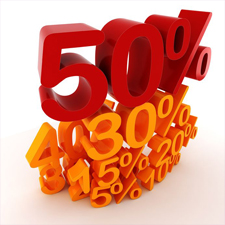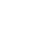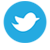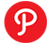Featured Listing:
StickerYou.com
Custom labels and decals for every aromatherapy practitioner need. Add custom professional look to your bottles, packaging and business. No restrictions in terms of quantity, shape and size - your needs and quality of our products is all that matters.

Essential Oil & Aromatherapy Resources:

# Calculating Percentages for Aromatherapy Recipes and Formulations

To work with aromatherapy and personal care formulations, it is essential to understand the following:

• How to calculate percentages and how to convert them to their decimal equivalents
• How to convert units of measure (pounds, ounces, gallons, fluid ounces, etc.)
• How to work with formulations that denote ingredients in "parts" of the total batch size

Calculating ingredient measurements may seem daunting at first, but it becomes straightforward as you work with more recipes. This guide will help get you started with all three of the above skills.

## Understanding Percentages

(Skip This Section if You Already Have a Strong General Understanding of Percentages)Often, aromatherapy and personal care recipes and formulations call for ingredients, such as essential oils, that are expressed as a percentage of the final batch size. It is important to understand how to accurately work with percentages, so let's start from the beginning...

A percentage is a number or ratio expressed as a fraction of 100. [This definition is courtesy of Wikipedia.org.]

When you see a number such as 10% denoted as a percentage, it is equivalent to 10 divided by 100 (0.10).

The easiest way to work with percentages is to start by converting the percentage to its decimal equivalent. You can then multiply the total batch size by the decimal equivalent to determine the total amount of the ingredient to include in your formulation. The examples in this guide refer to batch sizes given in weight measurements (i.e. pounds, ounces and grams), but these same principles apply to volumetric measurements (i.e. milliliters, fluid ounces and gallons). If a recipe or instruction does not specify whether to calculate by weight or volume, it is generally assumed that you will calculate by weight of the batch or sometimes the weight of a particular phase or portion of the recipe. For example, antioxidants are often added to soap at 0.10 to 2% of the weight of the oil phase.

### Percentage to Decimal Conversion Example:

• 10%: Let's start by converting 10% to its decimal equivalent. 10% is the equivalent of 10/100. When you divide 10 by 100, the result is 0.10.
• 20%: The same process holds true for any percentage that you need to convert to a decimal. 20%, for example, is equivalent to 20/100. When you divide 20 by 100, the result is 0.20.

Use the following tables as a resource for calculating most percentages that you'll come across. If you run into a more obscure percentage like 42%, simply divide 42 by 100 to get 0.42.

 Percentage: EquivalentFraction: ReducedEquivalentFraction: EquivalentDecimalValue 5% 5/100 1/20 0.05 10% 10/100 1/10 0.10 15% 15/100 3/20 0.15 20% 20/100 1/5 0.20 25% 25/100 1/4 0.25 30% 30/100 3/10 0.30 35% 35/100 7/20 0.35 40% 40/100 2/5 0.40 45% 45/100 9/20 0.45 50% 50/100 1/2 0.50

 Percentage: EquivalentFraction: ReducedEquivalentFraction: EquivalentDecimalValue 55% 55/100 11/20 0.55 60% 60/100 3/5 0.60 65% 65/100 13/20 0.65 70% 70/100 7/10 0.70 75% 75/100 3/4 0.75 80% 80/100 4/5 0.80 85% 85/100 17/20 0.85 90% 90/100 9/10 0.90 95% 95/100 19/20 0.95 100% 100/100 1/1 1.0

## Determining the Amount of Each Ingredient to Use in a Recipe When Ingredients Are Given as a Percentage

Let's suppose that you want to work with a basic balm recipe that includes the following ingredients. Let's also suppose that you want to make 5 pounds of the balm. If you're brand new to formulating, it is recommend that you start by making small trial batches, but this is intended to serve only as an introductory calculation example. To keep it as simple as possible, essential oils are not included in this initial example.

• 10% Beeswax
• 20% Jojoba
• 70% Shea Butter

### Step 1

When working with percentages, begin by referring to the total size of your batch and take note of the measurement unit (i.e. pounds, ounces, grams, ml) In this case, we're working with a total batch size that is denoted in pounds.

### Step 2

Start with the first ingredient, 10% Beeswax, and convert 10% to a decimal. 10% = 0.10.

### Step 3

Multiply the decimal equivalent for the Beeswax that you just calculated (.10) by the total batch size of 5 pounds: 5 pound batch size x 0.10 beeswax = 0.50 pounds.

Therefore, you will need 0.50 pounds (or half a pound) of Beeswax to make 5 pounds of this balm.

Use the same process to calculate the Jojoba and Shea Butter amounts needed for the recipe. Here are those calculations:

#### Jojoba

5 pound batch size x 0.20 (the equivalent of 20%) Jojoba = 1 pound. Therefore, 1 pound of Jojoba is needed to make 5 pounds of this balm.

#### Shea Butter

5 pound batch size x 0.70 (the equivalent of 70%) Shea Butter = 3.50 pounds. Therefore, 3.50 pounds of Shea Butter is needed to make 5 pounds of this balm.

## Understanding How to Convert Between Units of Measure

Sometimes, you may find yourself working with a recipe that requires that you work with very small or very large amounts of particular ingredients.

Let's suppose that you are now working with a different balm recipe that calls for the following ingredients. And let's say this time that you only want to make 2 pounds of the balm:

• 2% Helichrysum Essential Oil
• 8% Beeswax
• 20% Jojoba
• 70% Shea Butter

When working with small percentages of ingredients such as the 2% essential oil called for in the recipe, it can become challenging to try to accurately measure out .04 pounds of the essential oil (the amount called for - see above to confirm how to perform this calculation).

The alternative to trying to weigh small, awkward quantities such as .04 pounds of essential oil, is to first convert the batch size to a smaller unit such as ounces or grams.

The form of measurement that you are most comfortable with and the type of scale that you use will play a part in which measurement you select.

### Converting to Ounces

Let's assume that we have a scale that is capable of measuring in ounces and in fractions of an ounce. There are 16 ounces in a pound. Since the batch size is 2 pounds, the batch size is equivalent to 32 ounces (2 pounds x 16 ounces).

### Converting to Grams:

Let's assume that we have a small digital scale that is capable of measuring in grams. There are 453.60 grams in a pound. The batch size is 2 pounds, equivalent to 907.20 grams (2 pounds x 453.60 grams).

To determine the amount of Helichrysum Essential Oil needed for this example recipe in grams, multiply 0.02 (the equivalent of the 2% Helichrysum Essential Oil) by 907.20 grams: 0.02 Helichrysum Essential Oil x 907.20 grams = 18.10 grams of Helichrysum Essential Oil needed for this recipe (it's okay to round down to 18 grams).

## Understanding How to Work with Formulations That Denote Ingredients in "Parts" of the Total Batch Size

Sometimes, especially for essential oil diffuser recipes in which the blend consists of 100% essential oil, you will see measurements in a recipe denoted like this:

• 1 Part Spearmint Essential Oil
• 2 Parts Lemon Essential Oil
• 3 Parts Lavender Essential Oil

When working with recipes denoted in "parts," start by counting the total number of "parts" shown in the recipe. In this example, we have 1 part Spearmint Essential Oil plus 2 parts Lemon Essential Oil plus 3 parts Lavender Essential Oil. 1 + 2 + 3 = 6 total parts.

To calculate how much of essential oil that you need, you divide the number of parts shown for each ingredient by the total number of parts called for in the recipe (in this case, 6 total parts):

• 1 Part Spearmint Essential Oil = 1 part Spearmint Essential Oil divided by 6 total parts in the recipe = 0.17
• 2 Parts Lemon Essential Oil = 2 parts Lemon Essential Oil divided by 6 total parts in the recipe = 0.33
• 3 Parts Lavender Essential Oil = 3 parts Lavender Essential Oil divided by 6 total parts in the recipe = 0.50

Suppose that you want to work in ounces and that you just want to make 16 fl. ounces of this essential oil diffuser blend. (If you're new to holistic aromatherapy, I don't really recommend making large batches like this. This is merely a calculation example). Multiply the total batch size of 16 ounces with the calculated percentages derived above to determine how much of each ingredient is needed.

• 1 Part Spearmint Essential Oil = 1 Part Spearmint Essential Oil divided by 6 total parts in the recipe = 0.17 x 16 ounce total batch size = 2.72 ounces Spearmint Essential Oil needed
• 2 Parts Lemon Essential Oil = 2 parts Lemon Essential Oil divided by 6 total parts in the recipe = 0.33 x 16 ounce total batch size = 5.28 ounces Lemon Essential Oil needed
• 3 Parts Lavender Essential Oil = 3 parts Lavender Essential Oil divided by 6 total parts in the recipe = 0.50 x 16 ounce total batch size = 8 ounces Lavender Essential Oil needed

## Measurement Conversion Tools

The following Web sites offer measurement conversion tools that can help you convert between measurement types. We hope that you find these tools helpful. As these tools are supplied by third parties and outside Web sites, we cannot be responsible for the accuracy or usability of these tools.

Common Weight and Mass Conversions

Volume Conversions

## Check and Verify All Calculations

This article is intended for basic, general informational purposes only. Please doublecheck and verify all calications. AromaWeb is not responsible for any typos or calculation errors.

## Source

I originally wrote the above article for From Nature With Love. A special thank you to Anail Mitra, CEO of FNWL for warmly granting permission to include the article on AromaWeb. I've slightly modified the article to focus upon calculating essential oils. You can find the original article on FNWL here.Let's Stay Connected!